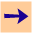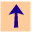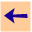7 Ask-the-user

The ask-the-user facility exists, but is poorly documented in this manual. The subgoals have to be ground before being asked of the user. This will be fixed in future implementations.

To to make an atom askable, you can issue the command:

```cilog:askable atom.
```
Whenever a ground instance of the atom is attempted to be proved, the user is asked if it is true. The system may ask:
```Is g true?
```
The user can reply with one of:
yes.
if g is known to be true. The system will not ask this instance again.
no.
if g is known to be false. The system will not ask this instance again.
unknown.
if g is unknown. In this case any applicable clauses for g can be used.
fail.
to fail the subgoal (but not record an answer). This is only used to test you axiomatization.
why.
to see why the system was asking this question. This then enters the why interaction described in Section 6.4.
prompt.
help.
Note the assumption that CILOG makes about the interaction between asking the user about g and using clauses for g. It assumes that if the user knows whether g is true, the user's answer should be used. Any rules for g are used only if the user doesn't know whether g is true or not.

Example. The following gives the example of the electrical example with askables (see , page 214):

```cilog: load 'cilog_code/ch6/elect_askable.pl'.7 Ask-the-user